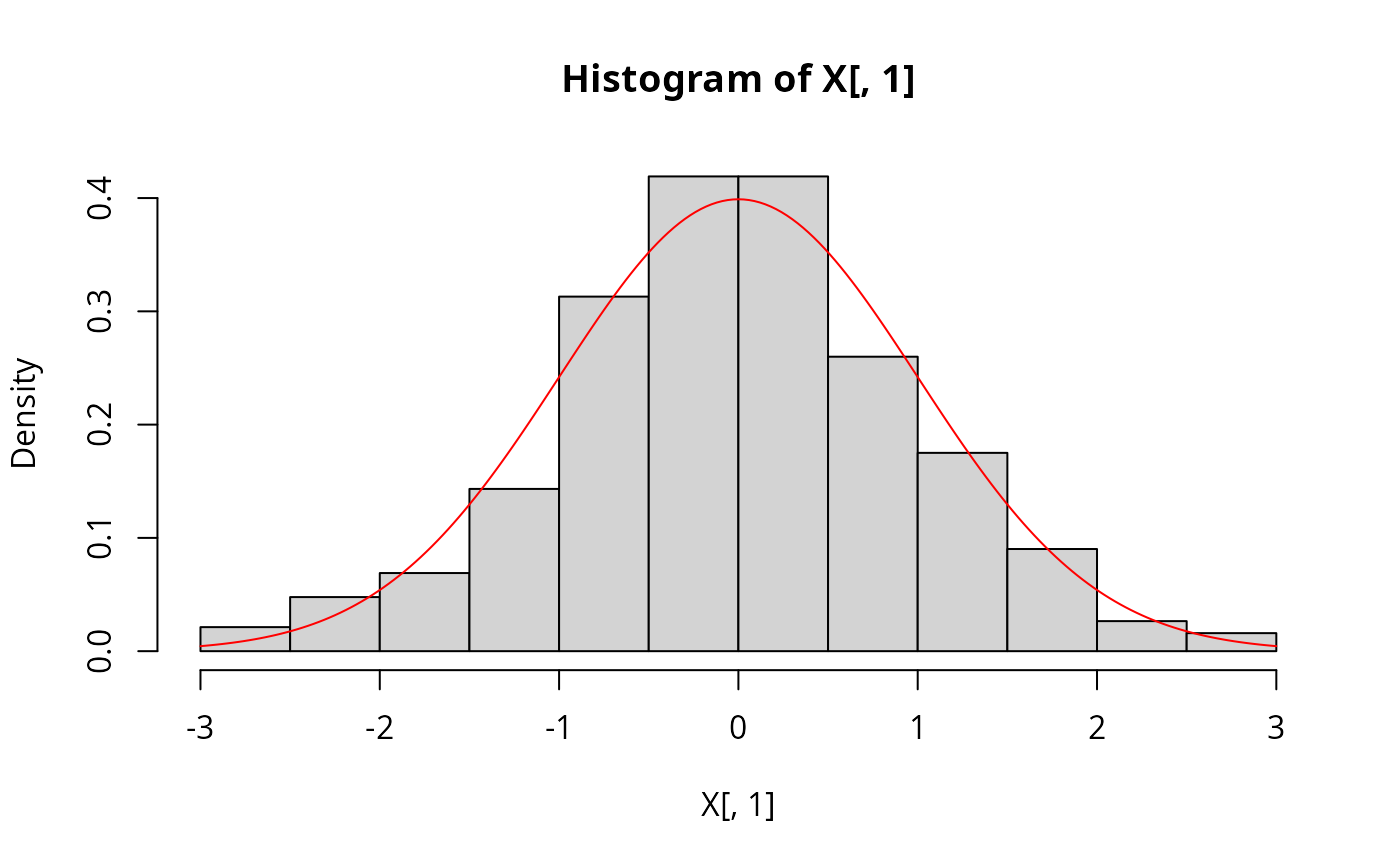Center and scale a "timeSeries" object.

## Usage

# S4 method for timeSeries
scale(x, center = TRUE, scale = TRUE)

## Arguments

x

an object from class "timeSeries".

center, scale

a numeric vector or a logical value, see ‘Details’.

## Details

scale centers and/or scales the columns of a "timeSeries" object.

The value of center determines how column centering is performed. If center is a numeric vector with length equal to the number of columns of x, then each column of x has the corresponding value from center subtracted from it. If center is TRUE then centering is done by subtracting the column means (omitting NAs) of x from their corresponding columns, and if center is FALSE, no centering is done.

The value of scale determines how column scaling is performed (after centering). If scale is a numeric vector with length equal to the number of columns of x, then each column of x is divided by the corresponding value from scale. If scale is TRUE then scaling is done by dividing the (centered) columns of x by their standard deviations if center is TRUE, and the root mean square otherwise. If scale is FALSE, no scaling is done.

## Value

a centered and/or scaled "timeSeries" object

## Examples

## Load Series:
x <- 100* LPP2005REC[, c("SBI", "SPI")]

## Scale and Center -
X <- scale(x)
hist(X[, 1], prob=TRUE)
s <- seq(-3, 3, length=201)
lines(s, dnorm(s), col="red")